Next: Peelle's Pertinent Puzzle Up: Use and misuse of Previous: Offset uncertainty   Contents

### Normalization uncertainty

Let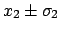and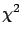be the two measured values, andthe common standard uncertainty on the scale: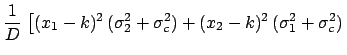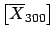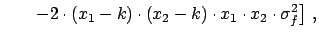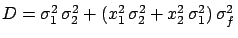(6.51)

where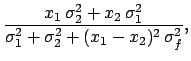. We obtain in this case the following result: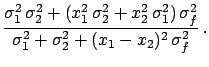(6.52)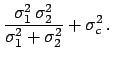(6.53)

With respect to the previous case,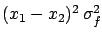has a new term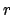in the denominator. As long as this is negligible with respect to the individual variances we still get the weighted average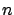, otherwise a smaller value is obtained. Calling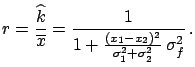the ratio betweenand, we obtain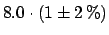(6.54)

Written in this way, one can see that the deviation from the simple average value depends on the compatibility of the two values and on the normalization uncertainty. This can be understood in the following way: as soon as the two values are in some disagreement, the fit starts to vary the normalization factor (in a hidden way) and to squeeze the scale by an amount allowed by, in order to minimize the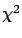. The reason the fit prefers normalization factors smaller than 1 under these conditions lies in the standard formalism of the covariance propagation, where only first derivatives are considered. This implies that the individual standard deviations are not rescaled by lowering the normalization factor, but the points get closer.
Example 1.
Consider the results of two measurements,and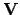, having a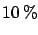common normalization error. Assuming that the two measurements refer to the same physical quantity, the best estimate of its true value can be obtained by fitting the points to a constant. Minimizingwith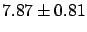estimated empirically by the data, as explained in the previous section, one obtains a value of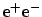, which is surprising to say the least, since the most probable result is outside the interval determined by the two measured values.
Example 2.
A real life case of this strange effect which occurred during the global analysis of the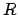ratio in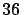performed by The CELLO Collaboration, is shown in Fig.. The data points represent the averages in energy bins of the results of the PETRA and PEP experiments. They are all correlated and the bars show the total uncertainty (see Ref.  for details). In particular, at the intermediate stage of the analysis shown in the figure, an overallsystematic error due theoretical uncertainties was included in the covariance matrix. Thevalues above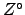GeV show the first hint of the rise of thecross-section due to the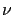pole. At that time it was very interesting to prove that the observation was not just a statistical fluctuation. In order to test this, themeasurements were fitted with a theoretical function having nocontributions, using only data below a certain energy. It was expected that a fast increase ofper number of degrees of freedom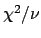would be observed aboveGeV, indicating that a theoretical prediction withoutwould be inadequate for describing the high-energy data. The surprising result was a repulsion'' (see Fig.) between the experimental data and the fit: Including the high-energy points with largera lower curve was obtained, while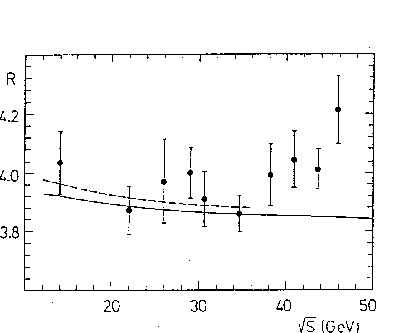remained almost constant.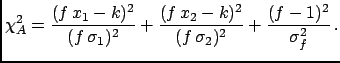To see the source of this effect more explicitly let us consider an alternative way often used to take the normalization uncertainty into account. A scale factor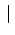, by which all data points are multiplied, is introduced to the expression of the:(6.55)

Let us also consider the same expression when the individual standard deviations are not rescaled: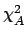(6.56)

The use of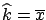always gives the result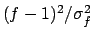, because the term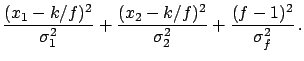is harmless6.9 as far as the value of the minimumand the determination onare concerned. Its only influence is on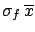, which turns out to be equal to quadratic combination of the weighted average standard deviation with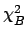, the normalization uncertainty on the average. This result corresponds to the usual one when the normalization factor in the definition ofis not included, and the overall uncertainty is added at the end.

Instead, the use of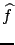is equivalent to the covariance matrix: The same values of the minimum, ofand ofare obtained, and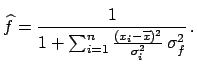at the minimum turns out to be exactly theratio defined above. This demonstrates that the effect happens when the data values are rescaled independently of their standard uncertainties. The effect can become huge if the data show mutual disagreement. The equality of the results obtained withwith those obtained with the covariance matrix allows us to study, in a simpler way, the behaviour of(=) when an arbitrary number of data points are analysed. The fitted value of the normalization factor is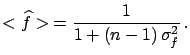(6.57)

If the values of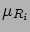are consistent with a common true value it can be shown that the expected value ofis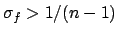(6.58)

Hence, there is a bias on the result when for a non-vanishinga large number of data points are fitted. In particular, the fit on average produces a bias larger than the normalization uncertainty itself if. One can also see thatand the minimum ofobtained with the covariance matrix or withare smaller by the same factorthan those obtained with.Next: Peelle's Pertinent Puzzle Up: Use and misuse of Previous: Offset uncertainty   Contents
Giulio D'Agostini 2003-05-15